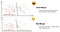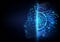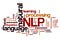# Machine Learning Quiz 03: Support Vector Machine

Let’s check your knowledge of Support Vector Machine. Here are 10 multiple-choice questions for you and there’s no time limit. Have fun!Image Source: A Top Machine Learning Algorithm Explained: Support Vector Machines (SVMs)

Question 1: What’s the objective of the support vector machine algorithm? (A) to find an optimal hyperplane in an N-dimensional space that distinctly classifies the data points where N is the number of features. (B) to find an optimal hyperplane in an N-dimensional space that distinctly classifies the data points where N is the number of samples. (C) to find an optimal hyperplane in an N-dimensional space that distinctly classifies the data points where N is the number…

# Machine Learning Quiz 02: Ridge, Lasso

Let’s check your basic knowledge of regularization techniques — Ridge and Lasso Regression. Here are 10 multiple-choice questions for you and there’s no time limit. Have fun!Source: google image

Question 1: For Ridge Regression, if the regularization parameter = 0, what does it mean?
(A) Large coefficients are not penalized
(B) Overfitting problems are not accounted for
(C) The loss function is as same as the ordinary least square loss function
(D) All of the above

Question 2: For Ridge Regression, if the regularization parameter is very high, which options are true? (Select two) (A) Large coefficients are significantly penalized (B) Can…

# How I learned NLP and What I learnedImage source: https://www.blumeglobal.com/learning/natural-language-processing/

I started my data science journey back in 2017. I did my first natural language processing project during my Master's program. Got the dataset from a Kaggle competition. The project was Determination of Toxic Comments and Analysis for the Minimization of Unintentional Model Bias. The primary goal of this project was to design a supervised and deep learning model that can perform binary classification in order to determine toxic and non-toxic comments from a social network. My course instructor was really happy with the outcome and gave me a LinkedIn recommendation.

In 2018, when I started my MS program I…

# Machine Learning Quiz 01: Linear Regression

Let’s check your basic knowledge of linear regression, the most commonly used machine learning algorithm. Here are 10 multiple-choice questions for you and there’s no time limit. Have fun!

Question 1: A linear regression model assumes “a linear relationship between the input variables and the single output variable.” What’s the meaning of this assumption?## Md Shahidullah Kawsar

Data Scientist at IDARE in the lone star state.